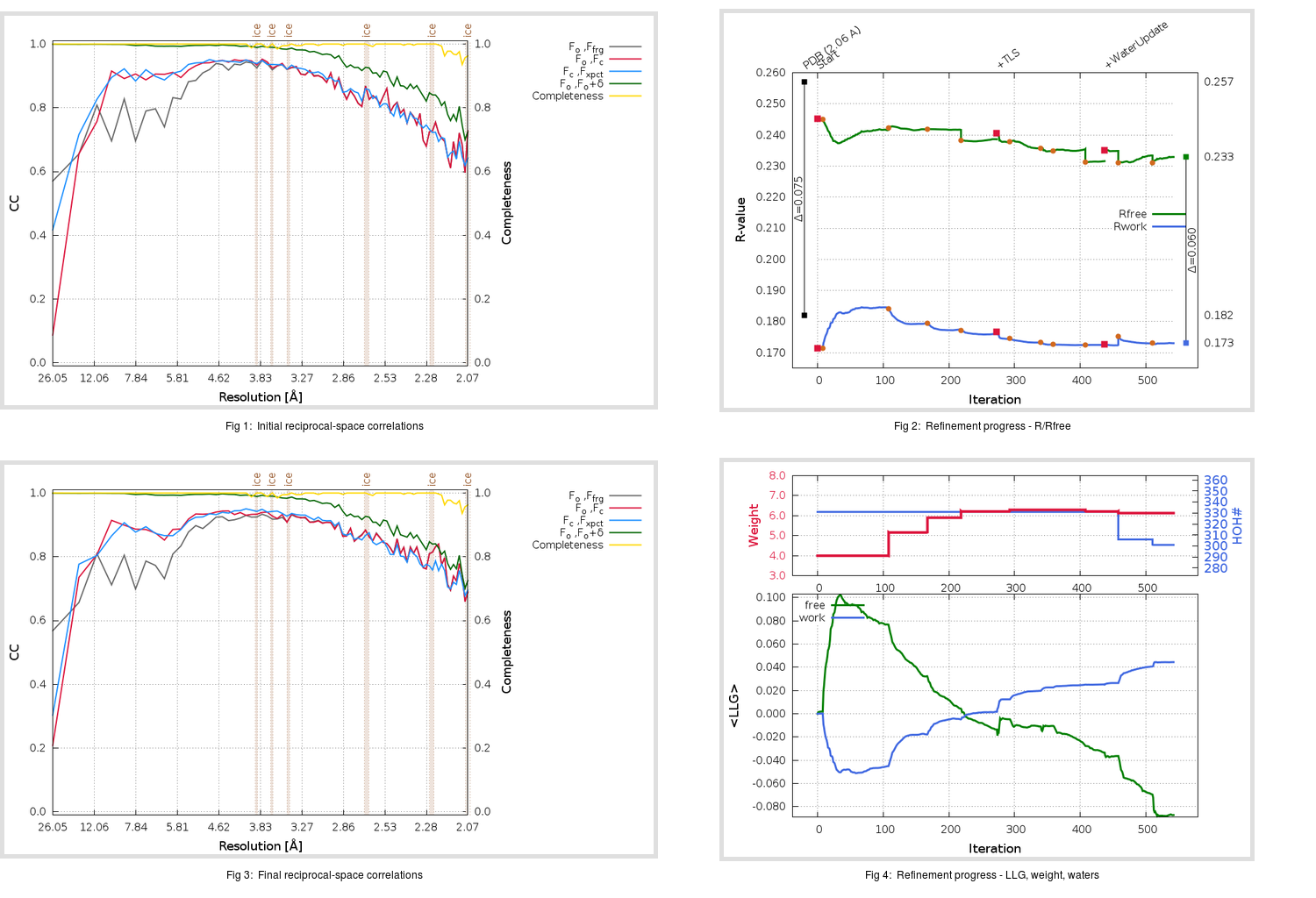Content:

## Deposited

` `
 Date deposited Date data collection Resolution R, Rfree 20200315 20200305 2.06 0.1780 0.2570

Molprobity (CCP4 7.0 version) summary:

```Ramachandran outliers =   0.33 %
favored =  97.35 %
Rotamer outliers      =   3.41 %
C-beta deviations     =     0
Clashscore            =   4.65
RMS(bonds)            =   0.0138
RMS(angles)           =   1.89
MolProbity score      =   1.77
Resolution            =   2.06
R-work                =   0.1780
R-free                =   0.2570
```

```Number of waters      =   331

<B> (all atoms) =   30.14 ( sd =   11.31 ) for       2731 non-hydrogen atoms
<B>   (protein) =   29.09 ( sd =   10.99 ) for       2370 non-hydrogen atoms
<B>     (water) =   36.70 ( sd =   10.48 ) for        331 non-hydrogen atoms
<B>    (others) =   43.41 ( sd =   21.39 ) for         30 non-hydrogen atoms

B min/max       (all non-hydrogen atoms) =   14.18 /   99.67
B min/max   (protein non-hydrogen atoms) =   14.18 /   92.81
B min/max     (water non-hydrogen atoms) =   14.99 /   99.67
B min/max     (other non-hydrogen atoms) =   30.55 /   73.72
```

## BUSTER (re-)refinement

` `

Molprobity (CCP4 7.0 version) summary:

```Ramachandran outliers =   0.33 %
favored =  98.34 %
Rotamer outliers      =   2.65 %
C-beta deviations     =     0
Clashscore            =   2.32
RMS(bonds)            =   0.0114
RMS(angles)           =   1.57
MolProbity score      =   1.33
Resolution            =   2.06
R-work                =   0.1731
R-free                =   0.2330
```

```Number of waters      =   301

<B> (all atoms) =   30.30 ( sd =    9.79 ) for       2701 non-hydrogen atoms
<B>   (protein) =   29.09 ( sd =    8.71 ) for       2370 non-hydrogen atoms
<B>     (water) =   38.90 ( sd =   12.08 ) for        301 non-hydrogen atoms
<B>    (others) =   41.84 ( sd =   20.00 ) for         30 non-hydrogen atoms

B min/max       (all non-hydrogen atoms) =   15.86 /  153.82
B min/max   (protein non-hydrogen atoms) =   15.86 /   66.97
B min/max     (water non-hydrogen atoms) =   15.96 /  153.82
B min/max     (other non-hydrogen atoms) =   31.61 /   65.17
```

Refinement progression:Results:

` `
 File Remark 5RFM_aB_refine.01_03_refine.pdb.gz exact refinement commands are in header 5RFM_aB_refine.01_03_refine.mtz.gz including original deposited data and several re-refinement map coefficients 5RFM_aB_refine.01_03_BUSTER_model.cif.gz including any non-standard compound restraints 5RFM_aB_refine.01_03_BUSTER_refln.cif.gz